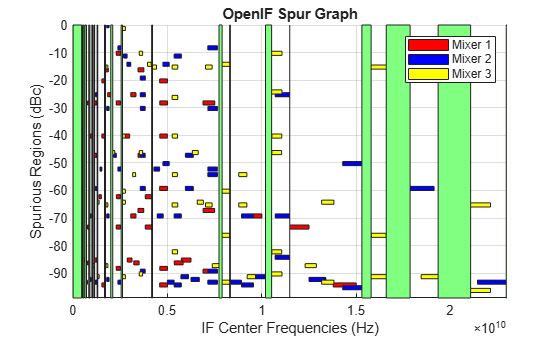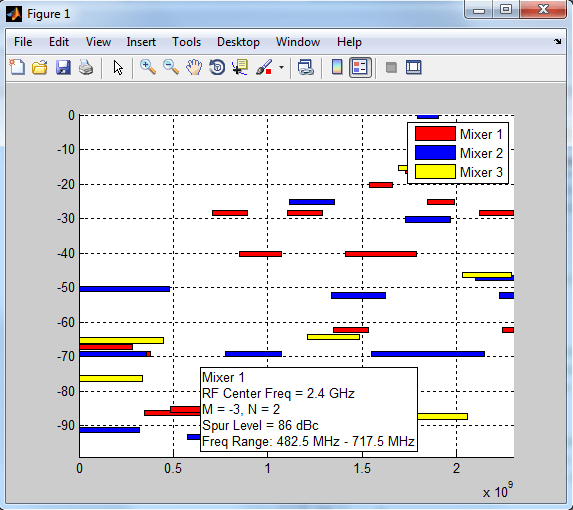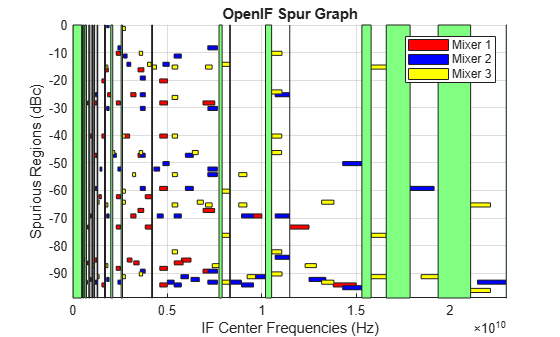# Finding Free IF Bandwidths

This example shows how to select an Intermediate Frequency (IF) that is free from any intermodulation distortion. First, you create an `OpenIF` object and specify whether you are designing a transmitter or receiver. Second, you use the `addMixer` function to define the properties of each mixer as well as the specific Radio Frequency (RF) it interacts with. Lastly, you view the results using the functions `report` and `show`.

### Background Knowledge (Mixer Spurs)

When converting from RF to IF (receiver) or from IF to RF (transmitter), a mixer is used. Unfortunately, mixers are nonlinear and their outputs contain energy at unwanted frequencies (we call these unwanted outputs "spurs"). The `OpenIF` tool helps you to select an IF which avoids having these spurious mixer outputs interfere with the mixer output. The output of the mixer is characterized by the following equation:

`${F}_{out}\left(N,M\right)=|N{F}_{in}+M{F}_{LO}|$`

where:

• ${F}_{in}$ is the input frequency.

• ${F}_{LO}$ is the local oscillator (LO) frequency.

• $N$ is a nonnegative integer.

• $M$ is an integer.

Only one of these output frequencies is the desired tone. For example, in a downconversion mixer (i.e. ${F}_{in}={F}_{RF}$) with a low-side LO (i.e. ${F}_{RF}>{F}_{LO}$), the case $N=1$, $M=-1$ represents the desired output tone. That is:

`${F}_{out}\left(1,-1\right)={F}_{IF}=|N{F}_{in}+M{F}_{LO}|={F}_{RF}-{F}_{LO}$`

All other combinations of $N$ and $M$ represent the spurious intermodulation products. To characterize these intermodulation products, an Intermodulation Table (IMT) is used.

### Background Knowledge (Intermodulation Tables)

The IMT provides information on the amount of power generated at each intermodulation product frequency. For accurate mixer spurs analysis results, the IMT should be built from simulated or measured data at the desired input signal and local oscillator frequency and power conditions. Extrapolation to other conditions will lead to inaccuracies.

Here is the IMT of a downconverting mixer with a low side LO, measured at ${F}_{in}={F}_{RF}=2.1$ GHz, ${P}_{in}={P}_{RF}=-10$ dBm, ${F}_{LO}=1.7$ GHz, and ${P}_{LO}=7$ dBm.

``` ! Element (N,M) gives power of |N*Fin+M*Flo| in dBc ! Top indices give M = ! Left-hand indices give N = %0 1 2 3 4 5 6 7 8 9 10 11 12 13 14 15 0% 99 26 35 39 50 41 53 49 51 42 62 51 60 47 77 50 1% 24 0 35 13 40 24 45 28 49 33 53 42 60 47 63 2% 73 73 74 70 71 64 69 64 69 62 74 62 72 60 3% 67 64 69 50 77 47 74 44 74 47 75 44 70 4% 86 90 86 88 88 85 86 85 90 85 85 85 5% 90 80 90 71 90 68 90 65 88 65 85 6% 90 90 90 90 90 90 90 90 90 90 7% 90 90 90 90 90 87 90 90 90 8% 99 95 99 95 99 95 99 95 9% 90 95 90 90 90 99 90 10% 99 99 99 99 99 99 11% 90 99 90 95 90 12% 99 99 99 99 13% 90 99 90 14% 99 99 15% 99 ```

Notice that it is a convention in industry-standard IMTs to assume symmetry, namely:

`${P}_{out}\left(N,M\right)={P}_{out}\left(N,-M\right)$`

and RF Toolbox™ software follows this convention.

If the measurement reveals that in fact the mixer is asymmetric, i.e.:

`${P}_{out}\left(N,M\right)\ne {P}_{out}\left(N,-M\right)$`

there is no way of accommodating this information in an industry-standard IMT. In this situation, the most common convention is to build an approximate model by placing the value:

`$\mathrm{max}\left({P}_{out}\left(N,M\right),{P}_{out}\left(N,-M\right)\right)$`

at position $N,M$.

Thus industry-standard IMTs in general and RF Toolbox in particular will over-estimate the power of one spur in each pair of asymmetric spurs.

In the IMT, a `0` always appears in the table at the position $N=1$, $M=1$, which represents both the desired signal and its symmetric image pair. All other entries are specified in dBc below the power of the mixer output at the desired frequency. (In the unlikely case of a spur being above the power of the desired, it will appear as a negative number, the magnitude of which is the spur power in dBc above the desired.)

For example, in the IMT above, at row $N=1$, column $M=3$, the IMT value is `13`. RF Toolbox will place a pair of symmetric IM products at:

`${F}_{out}\left(1,3\right)={F}_{in}+3{F}_{LO}$`

`${F}_{out}\left(1,-3\right)=|{F}_{in}-3{F}_{LO}|$`

each with a power level of -13 dBc. The absolute power of a spur in dBm is calculated by subtracting the IMT dBc value from the output power (also in dBm) of the desired tone.

By convention, the special value of `99` means the tone at that index is negligible.

### Design Requirements

Find a spur-free IF for a receiver. The receiver must be able to downconvert from three separate RF bands to the same (shared) IF. To find an IF center frequency that is spur-free for all three RF bands, your requirements must specify the RF Center Frequency, the RF Bandwidth, and the IF Bandwidth that goes with that particular RF:

```% RF band 1 RFCF1 = 2400e6; % 2.4 GHz RFBW1 = 200e6; % 200 MHz IFBW1 = 20e6; % 20 MHz % RF band 2 RFCF2 = 3700e6; % 3.7 GHz RFBW2 = 250e6; % 250 MHz IFBW2 = 20e6; % 20 MHz % RF band 3 RFCF3 = 5400e6; % 5.4 GHz RFBW3 = 250e6; % 250 MHz IFBW3 = 50e6; % 50 MHz ```

Next we must have an IMT measured for each RF band. Assume you have tested and measured the mixers you plan to use with the following results:

```IMT1 = [99 0 21 17 26; 11 0 29 29 63; 60 48 70 86 41; 90 89 74 68 87; 99 99 95 99 99]; IMT2 = [99 1 9 12 15; 20 0 26 31 48; 55 70 51 70 53; 85 90 60 70 94; 96 95 94 93 92]; IMT3 = [99 2 11 15 16; 27 0 16 41 55; 25 61 66 65 47; 92 83 66 77 88; 97 94 91 92 99];```

### Find Spur-Free frequencies using the OpenIF object

Create the object using the `OpenIF` function. Specify you are designing a receiver by setting the 'IFLocation' property to 'MixerOutput'.

`h = OpenIF('IFLocation', 'MixerOutput');`

Use the `addMixer` method to input the information for each RF band. Here low-side injection is assumed for each mixer, but high-side injection could be tried later.

```addMixer(h,IMT1, RFCF1, RFBW1, 'low', IFBW1); addMixer(h,IMT2, RFCF2, RFBW2, 'low', IFBW2); addMixer(h,IMT3, RFCF3, RFBW3, 'low', IFBW3);```

View the results textually using the `report` method.

`report(h);`
``` Intermediate Frequency (IF) Planner IF Location: MixerOutput -- MIXER 1 -- RF Center Frequency: 2.4 GHz RF Bandwidth: 200 MHz IF Bandwidth: 20 MHz MixerType: low Intermodulation Table: 99 0 21 17 26 11 0 29 29 63 60 48 70 86 41 90 89 74 68 87 99 99 95 99 99 -- MIXER 2 -- RF Center Frequency: 3.7 GHz RF Bandwidth: 250 MHz IF Bandwidth: 20 MHz MixerType: low Intermodulation Table: 99 1 9 12 15 20 0 26 31 48 55 70 51 70 53 85 90 60 70 94 96 95 94 93 92 -- MIXER 3 -- RF Center Frequency: 5.4 GHz RF Bandwidth: 250 MHz IF Bandwidth: 50 MHz MixerType: low Intermodulation Table: 99 2 11 15 16 27 0 16 41 55 25 61 66 65 47 92 83 66 77 88 97 94 91 92 99 There are no spur-free zones. The best attainable spur-free zone has a SpurFloor of 87. ```

View the results graphically using the `show` method.

```figure; show(h);```### Interpreting the Graphical Results

The figure created by the `show` method displays all relevant spurious frequency ranges as colored horizontal rectangles. If there any spur-free zones (there may not be) it will be displayed as vertical green rectangle.

In this example, as we can see in the figure, there are no spur-free zones. The legend in the upper right-hand corner tells us which color each Mixer is associated with. If we wish more detailed information about a spurious region, we can click on one of the rectangles:If we wish to find a spur-free zone, we will have to adjust some of the parameters of the setup.

### Adjusting a Mixer Property to find Spur-Free Zones

In the current setup, there are no spur-free zones available. We will need to adjust some of the setup parameters in order to find a spur-free zone. The values laid out in the design requirements (RF Bandwidth, RF Center Frequency, and IF Bandwidth) cannot be changed. However, some parameters (such as altering low- or high-side injection) are design decisions. We can see if changing the first mixer to high-side injection will open up a spur-free zone:

```h.Mixers(1).MixingType = 'high'; figure; show(h);```### Adjusting the SpurFloor to find Spur-Free Zones

If we wish to use low-side injection in all of the mixers, we must find acceptable spur-free zones by adjusting other parameters. Here we reset the OpenIF object to all low-side injection, and re-plot the results:

```h.Mixers(1).MixingType = 'low'; figure; show(h);```We notice there is a section around 500 MHz where there is a opening all the way down to roughly -85 dBc. We can find that zone by adjusting the `SpurFloor` property:

```h.SpurFloor = 85; show(h);```### References

 Daniel Faria, Lawrence Dunleavy, and Terje Svensen. "The Use of Intermodulation Tables for Mixer Simulations," Microwave Journal, Vol. 45, No. 4, December 2002, p. 60.